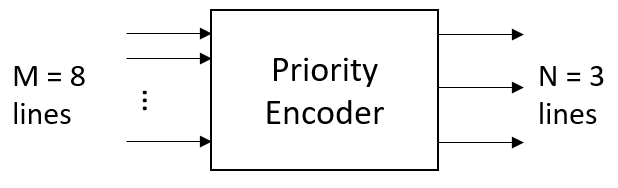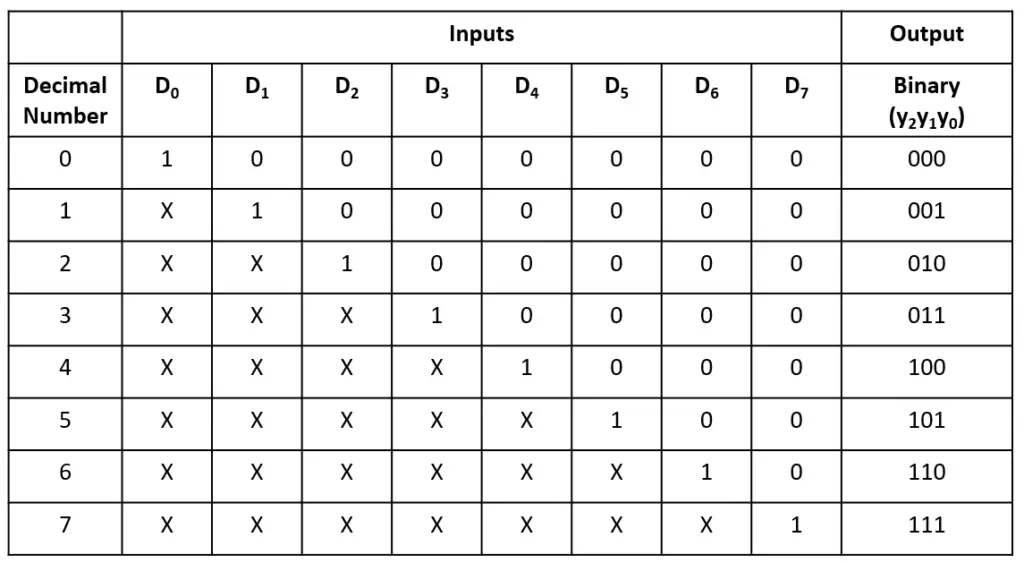Tutorials

The priority encoder overcome the drawback of binary encoder that generates invalid output for more than one input line is set to high. The priority encoder prioritizes each input line and provides an encoder output corresponding to its highest input priority.

The priority encoder is widely used in digital applications. One common example of a microprocessor detecting the highest priority interrupt. The priority encoders are also used in navigation systems, robotics for controlling arm positions, communication systems, etc.

## 8:3 Priority Encoder

### Block Diagram### Truth Table### 8:3 Priority Encoder Verilog Code

``````module priority_encoder(
input [7:0] D,
output reg [2:0] y);

always@(D) begin
casex(D)
8'b1xxx_xxxx: y = 3'b111;
8'b01xx_xxxx: y = 3'b110;
8'b001x_xxxx: y = 3'b101;
8'b0001_xxxx: y = 3'b100;
8'b0000_1xxx: y = 3'b011;
8'b0000_01xx: y = 3'b010;
8'b0000_001x: y = 3'b001;
8'b0000_0001: y = 3'b000;
endcase
end
endmodule``````

### Testbench Code

``````module tb;
reg [7:0] D;
wire [2:0] y;

priority_encoder pri_enc(D, y);

initial begin
\$monitor("D = %b -> y = %0b", D, y);
repeat(5) begin
D=\$random; #1;
end
end
endmodule``````

Output:

``````D = 00100100 -> y = 101
D = 10000001 -> y = 111
D = 00001001 -> y = 11
D = 01100011 -> y = 110
D = 00001101 -> y = 11``````

Verilog Codes